Question

A 3 kg box which is 3.9 cm by 3.9 cm by 19.4 cm long is dropped from a plane. What is the ratio of it's terminal velocity while falling with the narrow end down to the terminal velocity if it is dropped with the long end down. Assume that it has a coefficient of drag of 1.12 in either orientation.

Terminal velocity of a body is given by, V =(2mg/ρAc)^-2

where,

m is mass of the falling object =3 kg

g is the acceleration due to gravity. On Earth this is approximately 9.81 meters per second.

ρ is the density of the fluid the object is falling through= 3kg/ (3.9*3.9*19.4)*10^-6 m^3 = 10166.94 kg/m^3

A is the projected area of the object. This means the area of the object if you projected it onto a plane that was perpendicular to the direction the object is moving = (3.9*3.9)*10^-4 m^2 = 0.001521 m^2

C is the drag coefficient, here 1.12

therefore Terminal velocity, V= {(2*3*9.81)/(10166.94*0.001521*1.12)}^-2 = 1.84349 m/s

#### Earn Coins

Coins can be redeemed for fabulous gifts.

Similar Homework Help Questions
• ### Question 3 2 pts A 3 kg box which is 3.9 cm by 3.9 cm by...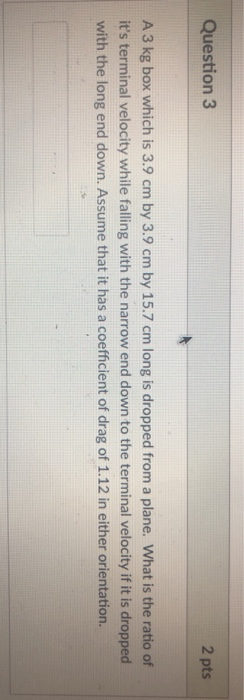Question 3 2 pts A 3 kg box which is 3.9 cm by 3.9 cm by 15.7 cm long is dropped from a plane. What is the ratio of it's terminal velocity while falling with the narrow end down to the terminal velocity if it is dropped with the long end down. Assume that it has a coefficient of drag of 1.12 in either orientation.

• ### 4. A 55 kg box rests on a horizontal surfcion betwce the box and the surface is 0.30, and the coe...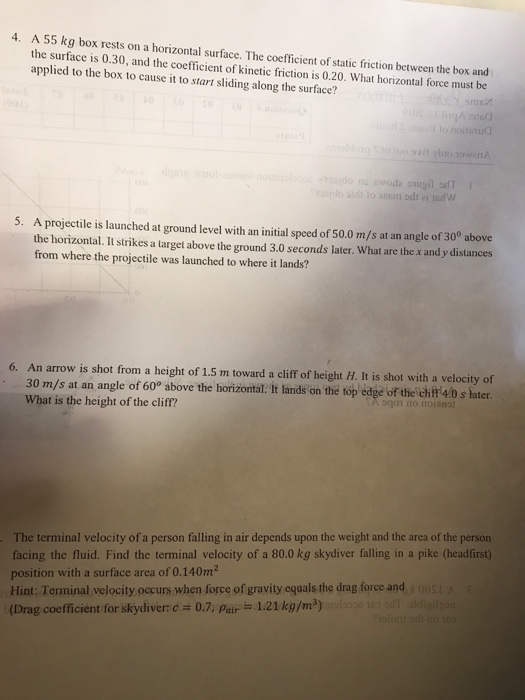#4 and #5!! 4. A 55 kg box rests on a horizontal surfcion betwce the box and the surface is 0.30, and the coefficient of kinetic friction is 0.20. What horizontal force must be applied to the box to cause it to start sliding along the surface? 4 :0 ovit 5. A projectile is launched at ground level with an initial speed of 50.0 m/s at an angle of 300 above the horizontal. It strikes a target above the ground...

• ### A 75 kg skydiver can be modeled as a rectangular "box" with dimensions 24 cm ×...

A 75 kg skydiver can be modeled as a rectangular "box" with dimensions 24 cm × 41 cm × 170 cm . If he falls feet first, his drag coefficient is 0.80. Part A What is his terminal speed if he falls feet first? Use ρ = 1.2 kg/m3 for the density of air at room temperature. Express your answer with the appropriate units.

• ### The large block shown is ?=18.0 cmx=18.0 cm wide, ?=22.0 cmy=22.0 cm long, and ?=25.00 cmz=25.00...

The large block shown is ?=18.0 cmx=18.0 cm wide, ?=22.0 cmy=22.0 cm long, and ?=25.00 cmz=25.00 cm high with a mass of 3.55 kg.3.55 kg. This block is passing through air (density of air ?air=1.43kg/m3).ρair=1.43kg/m3). Calculate the terminal velocity of the block if it is traveling downward with a drag coefficient of Γ=0.852Γ=0.852. See the hint panel for the drag force equation.

• ### 2. Suppose an object of mass 15 kg is dropped from a height near the surface...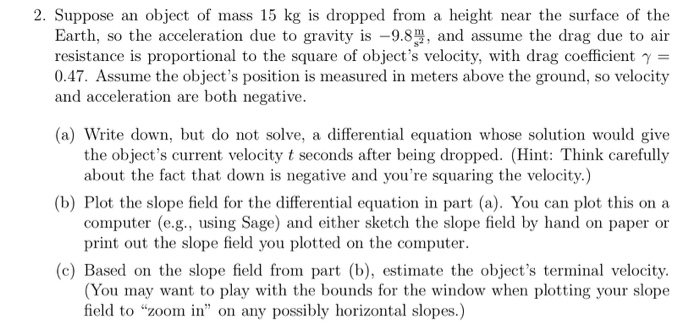2. Suppose an object of mass 15 kg is dropped from a height near the surface of the Earth, so the acceleration due to gravity is -9.8, and assume the drag due to air 0.47. Assume the object's position is measured in meters above the ground, so velocity (a) Write down, but do not solve, a differential equation whose solution would give resistance is proportional to the square of object's velocity, with drag coefficient γ and acceleration are both negative...

• ### A 60 g horizontal metal bar, 12 cm long, is free to slide up and down...

A 60 g horizontal metal bar, 12 cm long, is free to slide up and down between two tall, vertical metal rods that are 12 cm apart. A 0.080 T magnetic field is directed perpendicular to the plane of the rods. The bar is raised to near the top of the rods, and a 1.5 Ω resistor is connected across the two rods at the top. Then the bar is dropped. What is the terminal speed at which the bar...

• ### 7: A 60-kg box is placed against a uniform spring with a spring constant (k) of...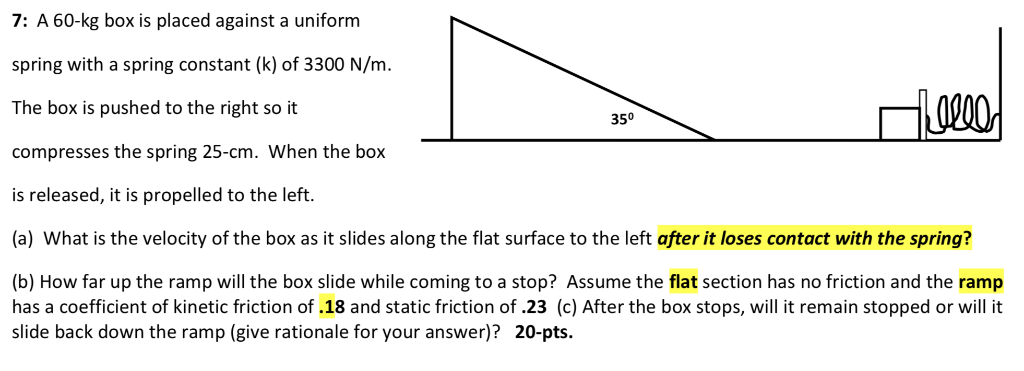7: A 60-kg box is placed against a uniform spring with a spring constant (k) of 3300 N/m The box is pushed to the right so it compresses the spring 25-cm. When the box is released, it is propelled to the left. (a) What is the velocity of the box as it slides along the flat surface to the left after it loses contact with the spring? 350 (b) How far up the ramp will the box slide while coming...

• ### A 55 g horizontal metal bar, 10-cm-long, is free to slide up and down between two...

A 55 g horizontal metal bar, 10-cm-long, is free to slide up and down between two tall, vertical metal rods that are 10 cm apart. A 5.0×10−2 T magnetic field is directed perpendicular to the plane of the rods. The bar is raised to near the top of the rods, and a 1.2 Ω resistor is connected across the two rods at the top. Then the bar is dropped. Part A What is the terminal speed at which the bar...

• ### A m = 10.0 kg box slides down a d = 00 m long ramp that...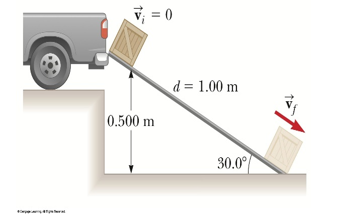A m = 10.0 kg box slides down a d = 00 m long ramp that is raised h = 0 .500 m above the ground (thus the ramp has a θ = 30.0⁰ incline). Assuming the ramp has a coefficient of kinetic friction of µk = 0.200 and the box is initially at rest, what is the final speed of the box when it makes it to the bottom of the ramp? [2.53 m/s] V = 0 d =...

• ### Three blocks (1,2,3) of mass 3.00 kg, 2.00 kg, 1.00 kg sit linearly 10.0 cm apart...

Three blocks (1,2,3) of mass 3.00 kg, 2.00 kg, 1.00 kg sit linearly 10.0 cm apart from each other on a frictionless surface. A 25 g bullet is shot at the first block, it travels through the first block, and embeds itself into the second block (without falling over) which then collides into the third block. a. Assuming the final collision is elastic, and the third block has a final velocity of 5.00 m/s, what is the velocity of block...